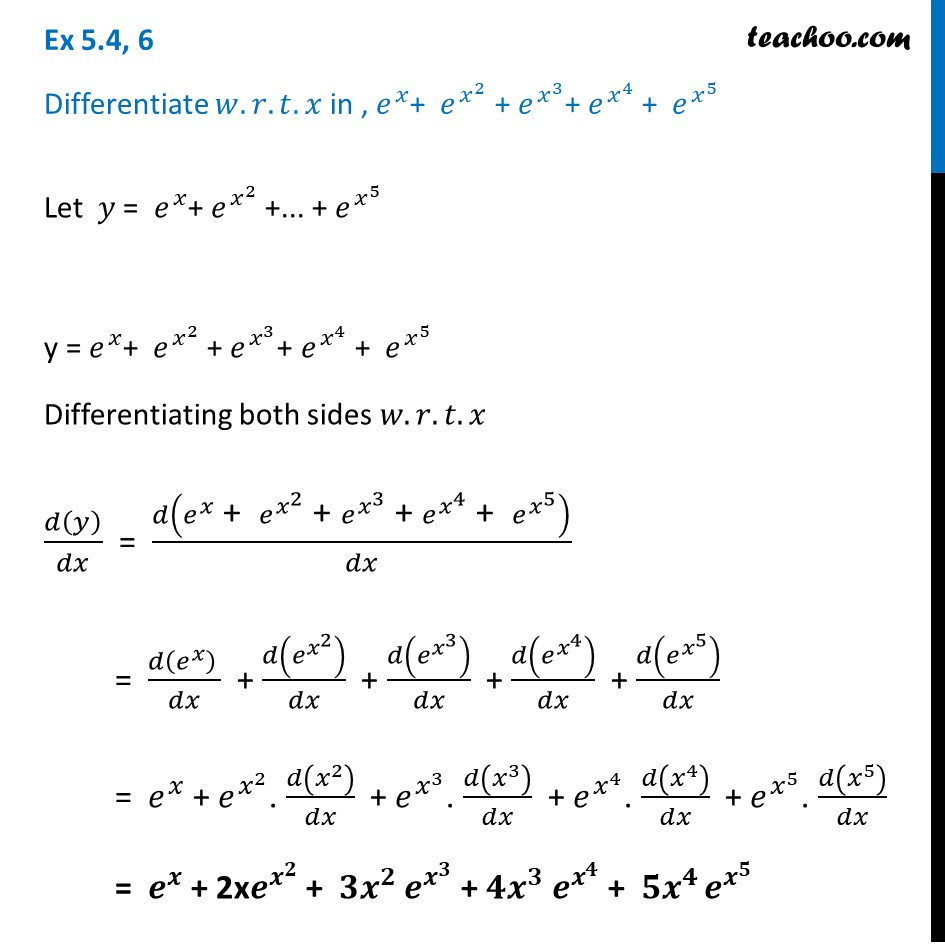Ex 5.4

Chapter 5 Class 12 Continuity and Differentiability
Serial order wiseLearn in your speed, with individual attention - Teachoo Maths 1-on-1 Class

### Transcript

Ex 5.4, 6 Differentiate 𝑤.𝑟.𝑡. 𝑥 in , 𝑒^𝑥+ 𝑒^(𝑥^2 ) + 𝑒^(𝑥^3 )+ 𝑒^(𝑥^4 ) + 𝑒^(𝑥^5 )Let 𝑦 = 𝑒^𝑥+ 𝑒^(𝑥^2 ) +... + 𝑒^(𝑥^5 ) y = 𝑒^𝑥+ 𝑒^(𝑥^2 ) + 𝑒^(𝑥^3 )+ 𝑒^(𝑥^4 ) + 𝑒^(𝑥^5 ) Differentiating both sides 𝑤.𝑟.𝑡.𝑥 𝑑(𝑦)/𝑑𝑥 = 𝑑(𝑒^𝑥 " + " 𝑒^(𝑥^2 ) " + " 𝑒^(𝑥^3 ) " + " 𝑒^(𝑥^4 ) " + " 𝑒^(𝑥^5 ) )/𝑑𝑥 = (𝑑(𝑒^𝑥 ) )/𝑑𝑥 + 𝑑(𝑒^(𝑥^2 ) )/𝑑𝑥 + 𝑑(𝑒^(𝑥^3 ) )/𝑑𝑥 + 𝑑(𝑒^(𝑥^4 ) )/𝑑𝑥 + 𝑑(𝑒^(𝑥^5 ) )/𝑑𝑥 = 𝑒^𝑥 + 𝑒^(𝑥^2 ). 𝑑(𝑥^2 )/𝑑𝑥 + 𝑒^(𝑥^3 ). 𝑑(𝑥^3 )/𝑑𝑥 + 𝑒^(𝑥^4 ). 𝑑(𝑥^4 )/𝑑𝑥 + 𝑒^(𝑥^5 ). 𝑑(𝑥^5 )/𝑑𝑥 = 𝒆^𝒙 + 2x𝒆^(𝒙^𝟐 ) + 𝟑𝒙^𝟐 𝒆^(𝒙^𝟑 ) + 𝟒𝒙^𝟑 𝒆^(𝒙^𝟒 ) + 〖𝟓𝒙〗^(𝟒 ) 𝒆^(𝒙^𝟓 )